# Root Locus for Control Systems

Publish Date: Nov 29, 2010 | 4 Ratings | 4.00 out of 5 | Print | Submit your review

## Overview

This tutorial shows how to plot the roots and the closed-loop response of a system, using LabVIEW and the LabVIEW Control Design and Simulation Module.

These tutorials are based on the Control Tutorials developed by Professor Dawn Tilbury of the Mechanical Engineering department at the University of Michigan and Professor Bill Messner of the Department of Mechanical Engineering at Carnegie Mellon University and were developed with their permission.

## Table of Contents

### 1. Closed-Loop Poles

The root locus of an open-loop transfer function H(s) is a plot of the locations (locus) of all possible closed loop poles with proportional gain k and unity feedback: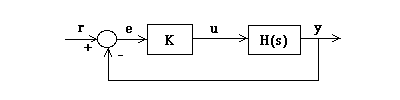Figure 1: Closed-Loop System

The closed-loop transfer function is: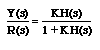Thus, the poles of the closed loop system are values of s such that 1 + K H(s) = 0.

If we use the relation H(s) = b(s)/a(s), then the previous equation has the form: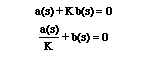Let n = order of a(s) and m = order of b(s) [the order of a polynomial is the highest power of s that appears in it].

We will consider all positive values of k. In the limit as k -> 0, the poles of the closed-loop system are a(s) = 0 or the poles of H(s). In the limit as k -> infinity, the poles of the closed-loop system are b(s) = 0 or the zeros of H(s).

No matter what we pick k to be, the closed-loop system must always have n poles, where n is the number of poles of H(s). The root locus must have n branches, each branch starts at a pole of H(s) and goes to a zero of H(s). If H(s) has more poles than zeros (as is often the case), m < n and we say that H(s) has zeros at infinity. In this case, the limit of H(s) as s -> infinity is zero. The number of zeros at infinity is n-m, the number of poles minus the number of zeros, and is the number of branches of the root locus that go to infinity (asymptotes).

Since the root locus is actually the locations of all possible closed loop poles, from the root locus we can select a gain such that our closed-loop system will perform the way we want. If any of the selected poles are on the right half plane, the closed-loop system will be unstable. The poles that are closest to the imaginary axis have the greatest influence on the closed-loop response, so even though the system has three or four poles, it may still act like a second or even first order system depending on the location(s) of the dominant pole(s).

### 2. Plotting the Root Locus of a Transfer Function

Consider an open loop system which has a transfer function of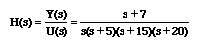How do we design a feedback controller for the system by using the root locus method? Say our design criteria are 5% overshoot and 1 second rise time.

### LabVIEW Graphical Approach

We can create a VI to plot the root locus, using the CD Root Locus VI from the Model Construction section of the Control Design palette.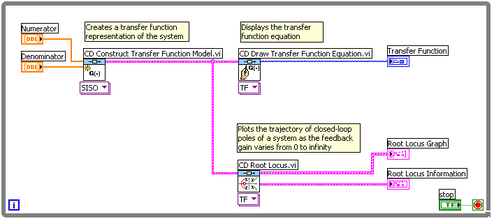Figure 2: Plotting Root Locus (Download)

### Hybrid Graphical/MathScript Approach

Alternatively, you can use a MathScript Node to plot the root locus, using the following code:

num=[1 7];

den=conv(conv([1 0],[1 5]),conv([1 15],[1 20]));

sys=tf(num,den);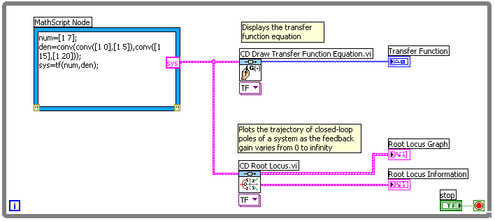Figure 3: Plotting Root Locus Using MathScript Node (Download)

### LabVIEW MathScript Approach

Yet another approach to this problem is to use the MathScript Window. Select Tools » MathScript Window, and enter the following code in the Command Window:

num=[1 7];

den=conv(conv([1 0],[1 5]),conv([1 15],[1 20]));

sys=tf(num,den);

rlocus(sys)

axis([-22 3 -15 15])

### Result

Using either the LabVIEW graphical approach, the LabVIEW MathScript approach, or the hybrid graphical/MathScript approach should return a plot similar to the one shown below in Figure 4.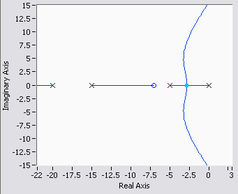Figure 4: Root Locus Plot

### 3. Choosing a Value of K from the Root Locus

The plot in Figure 4 above shows all possible closed-loop pole locations for a pure proportional controller. Obviously not all of those closed-loop poles will satisfy our design criteria.

To determine what part of the locus is acceptable, we can use the command sgrid on  to plot lines of constant damping ratio and natural frequency. In our problem, we need an overshoot less than 5% (which means a damping ratio Zeta of greater than 0.7) and a rise time of 1 second (which means a natural frequency Wn greater than 1.8).

Enter the command sgrid on in the MathScript command window and press Enter.

The following figure shows the plot that you should see. The red and green lines have been superimposed on the plot.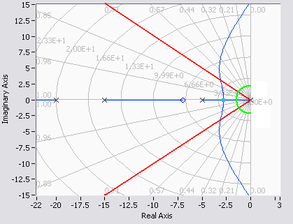Figure 6: Root Locus Plot with Grid Lines

On the plot above, the diagonal lines indicate constant damping ratios (Zeta), and the semicircles indicate lines of constant natural frequency (Wn). The red lines superimposed on the graph indicate pole locations with a damping ratio of 0.7. In between these lines, the poles will have Zeta > 0.7 and outside of the lines Zeta < 0.7. The green semicircle indicates pole locations with a natural frequency Wn = 1.8; inside the circle, Wn < 1.8 and outside the circle Wn > 1.8.

Going back to our problem, to make the overshoot less than 5%, the poles have to be in between the two red lines, and to make the rise time shorter than 1 second, the poles have to be outside of the green semicircle. So now we know only the part of the locus outside of the semicircle and in between the two lines are acceptable. All the poles in this location are in the left-half plane, so the closed-loop system will be stable.

From the plot above we see that there is part of the root locus inside the desired region. So in this case we need only a proportional controller to move the poles to the desired region.

You can use rlocfind command in the MathScript Window to choose the desired poles on the locus:

[k,poles] = rlocfind(sys)

Click and drag the closed-loop poles on the plot to designate where you want the closed-loop pole to be. You may want to select the points indicated in the plot below to satisfy the design criteria.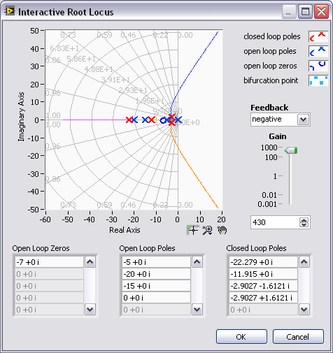Figure 7: Interactive Root Locus in MathScript Window

Note that since the root locus may have more than one branch, when you select a pole, you may want to find out where the other poles are. Remember they will affect the response too. From the plot above we see that all the poles selected are at reasonable positions. We can go ahead and use the chosen k as our proportional controller. Click OK to select these poles.

### 4. Closed-Loop Response

In order to find the step response, you need to know the closed-loop transfer function. You could compute this, or let LabVIEW do it for you in the MathScript Window.

sys_cl= feedback(k*sys,1)

The two arguments to the function feedback are the numerator and denominator of the open-loop system. You need to include the proportional gain that you have chosen. Unity feedback is assumed.

Finally, check out the step response of your closed-loop system.

step(sys_cl)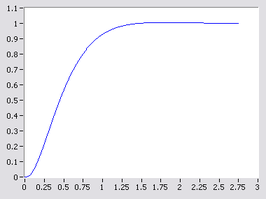Figure 8: Closed-Loop Response

As we expected, this response has an overshoot less than 5% and a rise time less than 1 second.

### Ratings

Rate this document

Answered Your Question?
Yes No